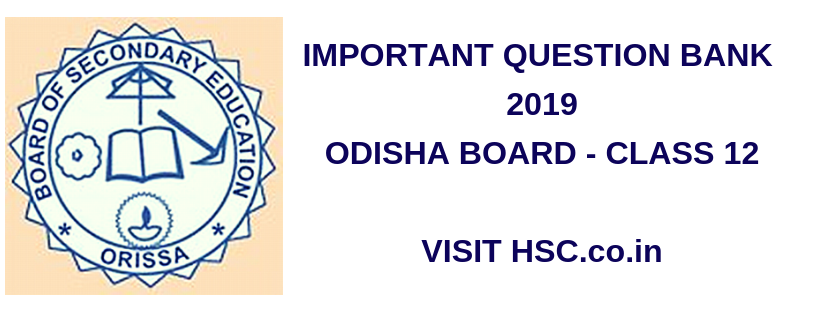# Physics – Important Question Bank for Odisha plus 2  class 12th (HSC) Board Exam 2019

HSC Board Exams are fast approaching and students are getting anxious about how to prepare for their HSC Board Exams. So we had mentioned some

After the tremendous success of our last year Important Question Bank for Odisha + 2 Board Exam 2016 and 2017 we have also created a list of  Most Important Question Bank for Odisha plus 2  class 12th (HSC) Board Exam 2017 which are likely to appear in HSC Board Exams this year.To unlock the content Click on any of 3 buttons available in the box below saying “This content is Locked”. Once you click on the button the content will get unlocked on same page itself. You must click on social media button showing in below box ie Facebook, Twitter or Google Plus to unlock the content.

Please use the comments box below and post questions that you think are important from your analysis. It would help the HSC community a lot.

Do subscribe to our updates so that you do not miss out on any important information that we push your way.

1. Rest transparent media A and B are separated by a plane boundary. The speeds of light are  Respectively in A and B. Then the critical angle for a ray of light for these two media is?
2. The minimum distance between an object and it’s real image formed by a conversation lens of focal length f is?
3. The average life if a radioactive sample is 2 years. It’s half-life in years is?
1. 1
2. 1.347
3. 1.386
4. 1.693
4. What is the rate of the efficiency of a hardware rectifier to that of a halfwave rectifier?
5. Satellite communication is brought about in ______ Hz frequency range.
6. Y-particles are not affected by a magnetic fields, but are affected by an electric field?
7. Two thin lenses of power 2D and -4D are kept in contact. What is the focal length of the combination in cm?
8. Define mobility of the charge carriers in a conductor.
9. Name the angle between the geographical meridian and the magnetic meridian of the Earth.
10. How much is the charge deposited on the capacitor of 10 micro ferret charged to a potential of 100 V ?

11. Whichever physical quantity is expressed in Tm/A ?

12. In Young’s double-slot experiment

Calculate the ratio of the intensities if the individual sources.

13. Draw a series combination of the resistors R1, R2 and R3.

14. Obtain the expression for the effective resistance in the combination of two resistors R1 and R2 in parallel. Sketch the combination also.

15. Inspire of the validity of ohms law it has some limitations. Give one limitation of ohms law.

16. Illustrate Biot-Savart law with necessary figure.

17. Compare Biot-Savart law with coloumb’s law for electrostatic field.

18. Give an expression for the magnetic field on the axis of a circular current loop.

19. State and explain Curie’s law.

20. Compare paramagnetism and ferromagnetism. Give examples of each.

21. Give an expression for the voltage and current in a transformer.

22. Explain resonance in an LCR circuit.

23. Draw a graphical representation of variation of current amplitude with frequency.

24. What do you mean by sharpness of resonance? Explain it.

25. Give a demonstration for total internal reflection.

26. Describe young’s experiment in interference with necessary theory.

27. Explain the refraction at a spherical surface using a schematic diagram.

28. In electrical magnetic spectra, the wave length and frequencies are inversely related. A radio can tune in to any station in the 7.5 MHz to 12 MHz band. Determine the corresponding wave length band.

29. Explain Einstein’s photo electric equation.

30. Name the different series of hydrogen atom.

31. Draw a typical illuminated P-n junction solar cell.

32. Obtain an expression for electric field for an electric dipole along its axis.

33. Derive an expression for equivalent emf and equivalent internal resistance when two  cells are connected in parallel.

34. Derive an expression for the magnetic field at a point along the axis of circular current  loop.

35. What is interference of light? Arrive at the conditions for constructive and destructive

interference by assuming the expression for intensity.

36. Derive an expression for total energy of an electron in hydrogen like atom assuming radius of the

orbit.

37. Explain the working of npn transistor as an amplifier in ce mode

38. Write the truth table and circuit symbol of a two-input NAND gate.

39. The distances of the real image of an object and the object, from the focus of a concave mirror are respectively b and a. Find the focal length of the concave mirror in terms of a and b.

40. Explain the working principle of step-up transformer.

41. Define the dimension of the resistivity of a material.

42. A plane mirror and a convex mirror are at distances of 20cm and 25cm respectively from an object determine the focal length of the convex mirror is the images formed by both the mirrors are at the same location without parallax.

43. The wavelength of a photon is equal to the de Broglie wavelength of a particle moving with a velocity v. Find the ratio of the energy of the photon to the kinetic energy of the particle.

44. Derive the relation between the electric field intensity and the potential gradient due to a point charge at a point in it’s field.

45. Define the lens Maker’s formula.

46. Derive the expression for the capacity of a parallel-plate capacitor filled with a dielectric.

Don’t forget to read : MUST REMEMBER THINGS on the day of Exam for HSC Students

## 4 thoughts on “Physics – Important Question Bank for Odisha plus 2  class 12th (HSC) Board Exam 2019”

1.biswa prakash das says:

ruther ford ‘s atom model

2.goutam raita says:

plez important quetions chemistry

1.goutam raita says:

plez chemistry important quetions 2019

3.Satyajit Naik says:

Imrotant quetions of math